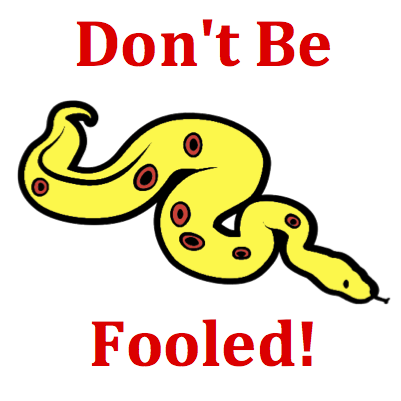# Momentum and Collisions - Mission MC4 Detailed HelpIn a physics lab, two carts undergo a collision on a low-friction track. Cart A has twice the mass and twice the speed of Cart B. It is assumed that the two carts collide in an isolated system. During the collision, Cart B (or Cart A) encounters ____ force, ____ impulse, ____ momentum change, and ____ acceleration.Newton's Third Law: In any interaction between two objects, the objects mutually exert forces upon each other. These forces are equal in magnitude and opposite in direction. So if object 1 exerts a force of F2 on object 2, then object 2 exerts an equal force (in the opposite direction) of F1 on object 1. In equation form   F1 = - F2The logic begins with Newton's third law (see Know the Law section). In a collision between any two objects - objects 1 and 2 (where 1 may refer to the golf ball and 2 refers to the more massive golf club) - the forces experienced by the objects are equal in magnitude. That is,    F1 = - F2 Common sense would tell us that these forces endure for the same amount of time. After all, if the forces result from a contact interaction, the ball cannot be contacting the club for a different amount of time than the club is contacting the ball. So     t1 = t2 Mathematical logic applied to the two equations above would lead to the conclusion that the product of F and t for object 1 is equal in magnitude (and opposite in direction) to the product of F and t for object 2. That is,    F1 • t1 = - F2 • t2 The above statement means that each object - golf ball and golf club - encounters the same impulse, directed in opposite directions. Finally, the impulse is equal to momentum change (see Know the Law section). Applied to this collision, one can conclude that the golf club and golf ball also experience the same momentum change. That is    m1 • ∆v1 = - m2 • ∆v2 In conclusion: in a collision between any two objects, the forces exerted on the objects are equal in magnitude enduring for the same amount of time to produce an equal impulse for each object and resulting in an equal momentum change for each object.Momentum Change - Impulse Theorem: When a force is exerted upon an object in a collision, the object is said to have encountered an impulse. The impulse is simply the mathematical product of the force exerted on the object and the amount of time over which it was exerted. The impulse changes the object's momentum and is equal to the amount of momentum change. Impulse = Momentum ChangeIn a collision between two objects, there are some quantities that are always the same for each object (collision force, collision time, impulse and momentum change) and some quantities that often vary (mass, velocity change and acceleration). A careful student of Physics will keep these quantities straight in their mind. Don't be fooled! While the force, impulse and momentum change are the same for each object, the velocity change and acceleration will be greatest for the least massive object.